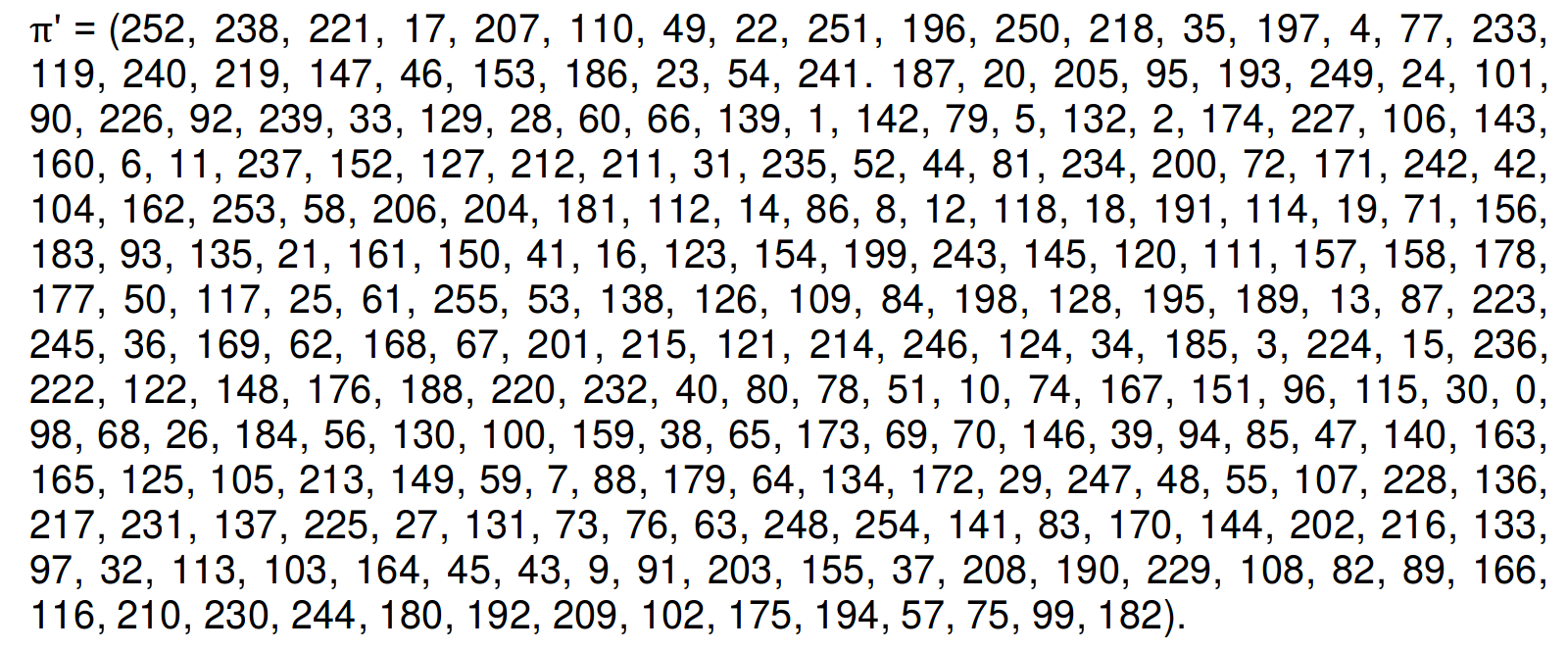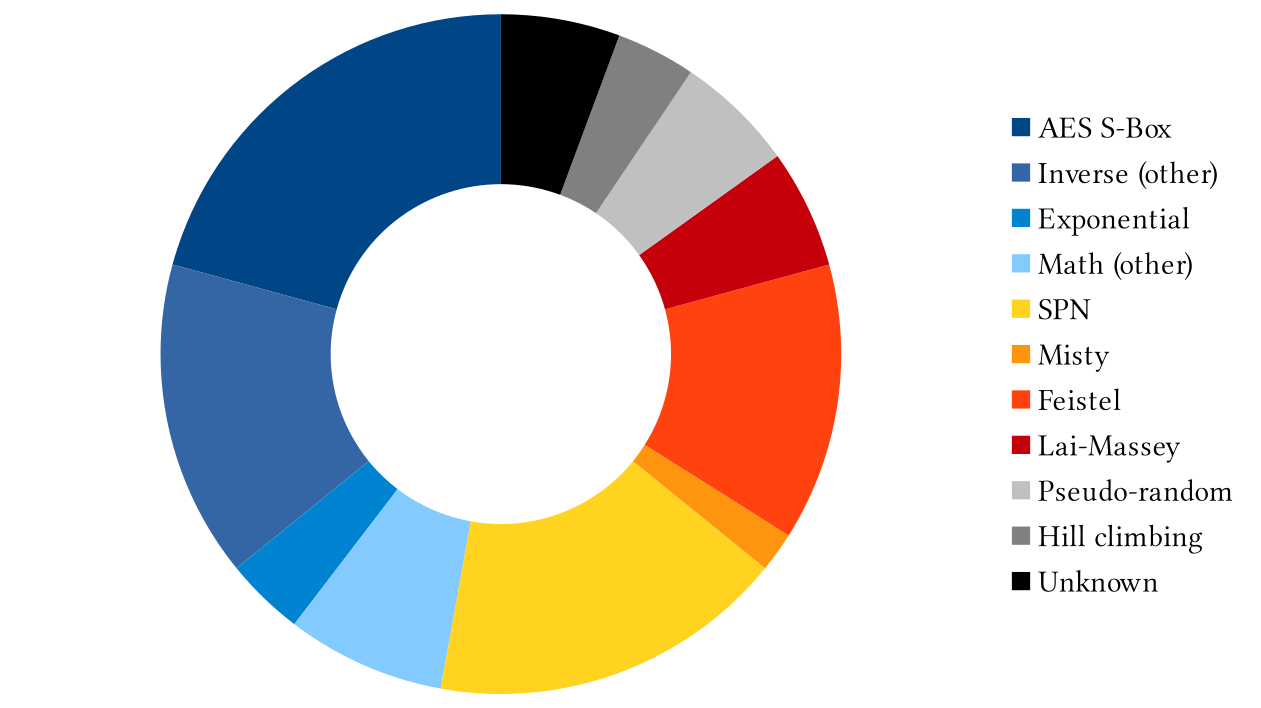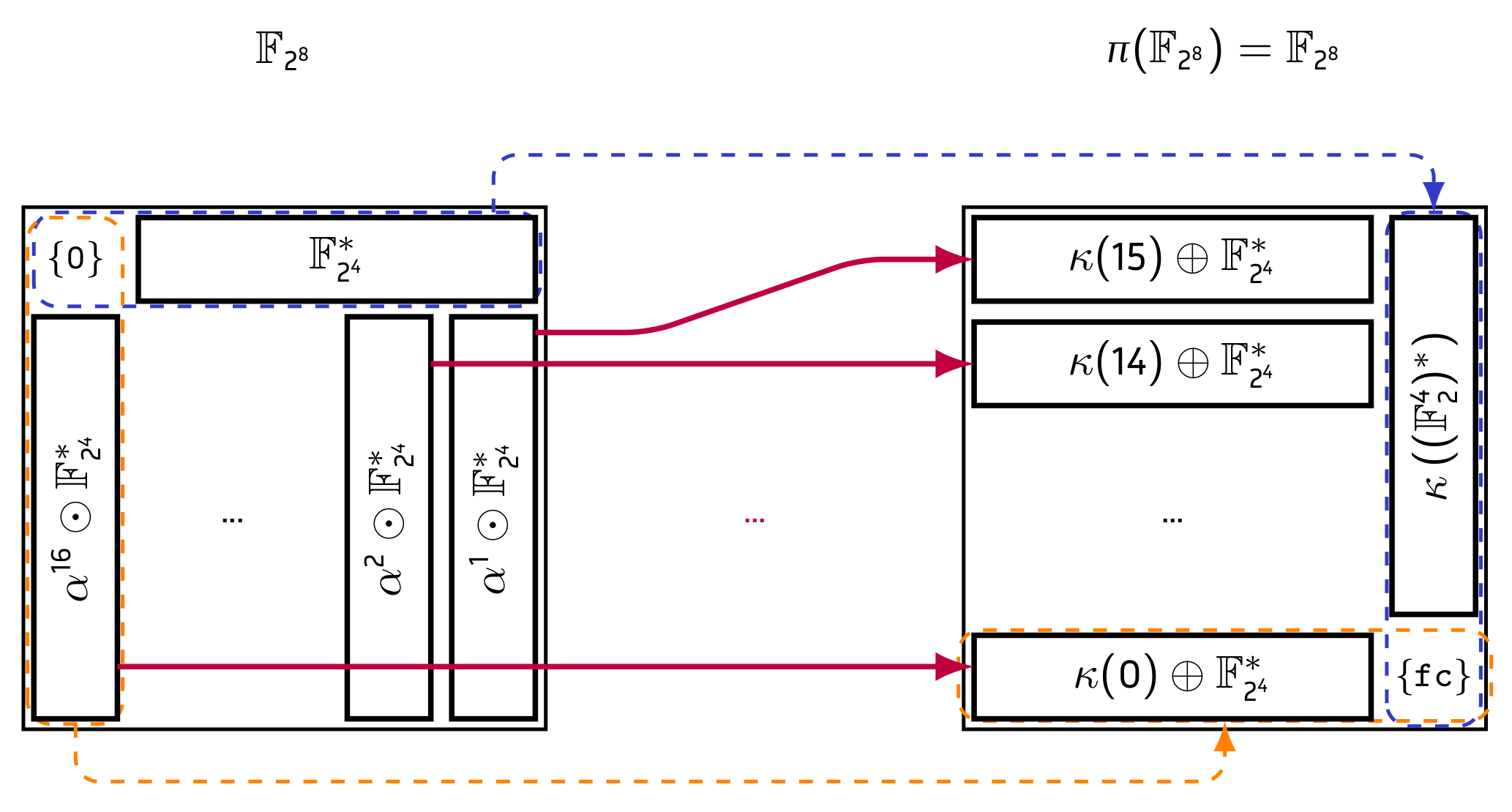inria.fr Go back Open original

# On the S-Box of Streebog and Kuznyechik

By Author: Leo Perrin

Recently, I have obtained new results  about the S-box used by both Russian standards in symmetric cryptography, namely the block cipher (Kuznyechik) and the hash function (Streebog). They are both GOST standards and RFCs, (respectively RFC 6986 and RFC 7801). In this page, I summarize these latest results using an FAQ. I encourage the interested reader to have a look at the actual research paper, which is available online (without any paywall!) on the ToSC website.

Cryptography is the science dealing with information security: how can we communicate in such a way that an eavesdropper cannot spy on us? As communications are done via computers, the techniques enabling such protections are algorithms that prevent attackers from accessing the information using a key: unless the key is known, it is impossible to read/modify the data. When the same key is used to process the information before sending and after receiving, we talk about symmetric cryptography.

It is necessary to continuously analyse these algorithms as they may eventually turn out to have flaws. This threat is not theoretical as cryptographic algorithms have failed in this way in the past. To take a recent example, the hash function SHA-1 was found to yield collisions, a critical failure for such an algorithm .

The algorithms that are used are usually those that have been standardized by some standardizing body (e.g. the American NIST, ISO/IEC, etc.). Each standardizing has its own internal process for choosing ciphers.

This process can be completely opaque as evidenced by the standardization of the DUAL_EC PRNG by the American NIST. This algorithm turned out to have been imposed by the NSA and to likely contain a backdoor, as detailed in . In this case, the algorithm was standardized basically because the NSA said it had to be. It is not a unique instance: the Russian algorithms discussed on this page were also designed secretly and then standardized without any competition. It is obviously very difficult to trust algorithms standardized in this fashion: where is the third party analysis? Where are the designers' explanations?

In order to foster trust, a much better strategy consists in organizing open competitions. For instance the AES and hash function NIST competitions yielded the standardization of algorithms that are now well trusted. This trust comes from the analysis received by these algorithms before they were standardized and from the quality of the theoretical frameworks used to construct them, frameworks which were carefully explained by their designers.

The AES, SHA-3 and the DUAL_ EC algorithms were all standardized by the same institution (the NIST), meaning that assessing the quality of a standard is therefore not as simple as looking at its origin.

Streebog is a cryptographic hash function. It was standardized by the Russian Federation in 2012. Kuznyechik is a block cipher which was standardized by the same country in 2015.

Both are intended to be used in Russia. At the time of writing, their authors are also pushing for their standardization by ISO/IEC.

One of the basic building block to construct symmetric algorithms is the block cipher. For example the AES, one of the most widely used and well studied symmetric cryptographic algorithms, is a block cipher. So is Kuznyechik. It is also common to use a block cipher to construct a hash function using a block cipher: it is the case of SHA-2, a very common hash function, and of Streebog.

Block ciphers are built by iterating a so-called round function whose goal is to provide a weak version of the properties that the block cipher is expected to fulfil. By iterating these rounds, the block cipher will achieve them. A key property is non-linearity. In practice, it is usually provided by a small component called S(ubstitution)-box. An S-box is a function operating on a small enough input that its output can be specified by its lookup table. The round function then contains the parallel application of said S-box over the whole state.

The Russian algorithms both use the same S-box, π. It is specified via its lookup table (Figure 1).Figure 1: The lookup table ofas it appears in its specification . This function maps 0 to 252, 1 to 238, …, and 255 to 182.

As they are the only source of non-linearity, the cryptographic properties of the S-box play a crucial role in the security argument given by the algorithm designers. In fact, designers are expected to explain how the S-box they used was designed and why they chose the structure their S-box has. For example, the AES has an S-box which is based on the multiplicative inverse in the finite field. This choice is motivated by the fact that both the linearity and the differential uniformity 1 of this permutation are the lowest known to be possible.

While the multiplicative inverse, and in fact the very S-box of the AES, are popular choices for constructing 8-bit S-boxes, they are not the only possiblity. I made a survey of the literature and compiled a list of all the 8-bit S-boxes I could get my hands on2 during my PhD . They are sorted by structure in Figure 2. As we can see, the structures used vary a lot, from clear mathematical description to block cipher like structures. These differences correspond to different goals. While it is necessary to have good cryptographic properties, it is also essential that the S-box be easy to implement for example in hardware. Depending on the priority of these constraints, the designers will use different structures.Figure 2: A survey of the structures to build the 8-bit S-boxes appearing in more than 50 different symmetric algorithms (from my PhD ).

In order to benefit from the easy implementation allowed by a given structure, it is necessary that the structure be public. More importantly, in order for cryptographers to be able to analyse the cipher properly, it is necessary that they are given all the information available and in particular the structure used to construct the S-box. It could be that the S-box interacts in an undesirable way with other components of the cipher and this interaction can only be noticed if the structure is known.

To see an example of the kind of constraints and trade-offs that naturally occurr when designing an S-box, you can have a look at at this e-mail sent to the CFRG mailing list by one of the designers of the Belarussian standard, BelT. He explains why they decided to choose an S-box based on a discrete exponentiation—a choice I personally see nothing wrong with.

The authors of Streebog and Kuznyechik have provided little to no information about their design process. Several cryptographers have tried to ask them about the structure of their S-box during conferences. The usual answer was equivalent to saying that they were picked at random from some set. They also claim to have lost the generation algorithm. Given the importance of the S-box, this loss should be worrying in and on itself.

With my co-authors Aleksei Udovenko and Alex Biryukov, we first identified two different decompositions of π which were published at two peer-reviewed venues: the conference EUROCRYPT'16 for the first  and the IACR Transactions on Symmetric Cryptology for the second . However, below, I will mostly talk about the third decomposition which has been published3 in a later edition of the IACR Transactions on Symmetric Cryptology . It is based on a structure which I called TKlog.

The TKlog, which I named after the TK26 (Technical Committee 26, the designers of the Russian algorithms), is a special family of permutations of whichis a member. These are defined by composing a discrete logarithm (mapping finite field elements to integers) with a strange but simple permutation mapping the integers back to finite field elements.

My claim that π has a TKlog structure is not up for debate: the unconvinced reader can simply run the small SAGE script below and see that it does indeed generate the right lookup table. It also shows the general TKlog structure: simply change cstt, lambda_vectors or s to generate another TKlog instance4

#!/usr/bin/sage from sage.all import * # arithmetic machinery
N = 8
X = GF(2).polynomial_ring().gen()
F = GF(2**8, name="a", modulus=X**8+X**4+X**3+X**2+1)
alpha = F.gen()
xor = lambda x,y : Integer(x).__xor__(Integer(y)) # arbitrary components
s = [0, 12, 9, 8, 7, 4, 14, 6, 5, 10, 2, 11, 1, 3, 13]
lambda_vectors = [0x12, 0x26, 0x24, 0x30]
cstt = 0xFC # subfunction
def kappa(x): result = 0 for j in xrange(0, 4): if (x >> j) & 1 == 1: result = xor(result, lambda_vectors[j]) return xor(result, cstt) # generating pi
# -- pi
pi = [kappa(0)]
# -- pi[x] for x > 0
for x in xrange(1, 2**N): l = int(F.fetch_int(x)._log_repr()) i, j = l % 17, floor(l / 17) if i == 0: y = kappa(16-j) else: gf_elmt = (alpha**17)**s[j] y = xor(kappa(16-i), gf_elmt.integer_representation()) pi.append(y) print pi


In this context, a decomposition is simply an algorithm that, when input, will return. This algorithm has a priori no reason to be unique. For example, the functiondefined over the integers, can be written as the composition ofandor as the composition ofand. In the case of, it is much, much, much more complicated, but at its core the situation is the same: there are multiple ways of computing it that all yield the same result.

Good question: since there are multiple ways of computing this function, why would the TKlog be the one originally intended by its designers?

This third decomposition is by far the simplest in terms of mathematics: it is completely described by a simple system of three equations, unlike the others. It also requires the fewest "arbitrary" constants; in fact, there are only aboutdifferent TKlog instances operating on 8 bits. This number is astonishingly small compared to the total number of 8-bit permutations, namely. Hence, the probability for a random 8-bit permutation to be a TKlog is around.

Let's put this number into perspective. The probability of winning the French lotery5 is around. Thus, the probability of obtaining a TKlog by picking an 8-bit permutation at random is comparable to the probability of winning the French lotery 66 times in a row!

If someone were to win this lotery 66 times in a row, would you conclude that they are astonishingly lucky or that some shenanigans were involved when picking the "random" numbers? The latter is the natural conclusion. Using the same reasoning, I claim that the presence of this structure inis intentional.

One of the only claim made by the designers of these algorithms is that they picked their S-box at random in some set of S-boxes. If they picked the parameters of the TKlog randomly, wouldn't their design technically match this description?

In my opinion, no.

Suppose that someone tells you that they are going to choose 5 numbers betweenandrandomly. To your surprise, they foundand. When confronting them about the obvious pattern in their result, they tell you that they used 6-sided dice rolls (i.e., a random process) to generate these numbers and that they are indeed betweenand. In this case, your friend left out so much information about their process that the little given was essentially meaningless, if not misleading.

Furthermore, the authors of Streebog have argued in informal discussions (see a summary in ) that they wanted to avoid having too strong an algebraic structure in order to prevent some attacks. While there is nothing wrong with this approach a priori, it is completely at odds with their final result: as shown in the next section, their S-box has an extremely strong algebraic structure.

Any function operating onbits can be written as a function over a finite field. The exact correspondance between the finite field elements and their binary representation is not unique; it is determined by a specific polynomial. In the case of, this field is. It contains, as a subset, the finite field. As these sets are fields, we can define an addition (which here corresponds to a XOR, denoted "") and a multiplication6 (denoted "").

The S-box(as well as all TKlogs) maps the subsetofto a subset of the form. This phenomenon is summarized in Figure 3. This means thatmaps a simple partition of its input to a simple partition of its output.

Even worse, allcan be written7 aswithand we then have that ifthenfor some functionsand. In other words, the output can divided into two halves, each of which depends only on one half of the input. However, in the input, the definition of these halves is not linear. As an other consequence, the wayacts on each subsetofforis always the same. Note also thatis a permutation of, meaning that its output is also in this subset.Figure 3: The main property of: it maps a simple partition of its input to a simple partition of its output.

First of all, that is very strong algebraic structure where they previously claimed that there was none.

Second, Arnaud Bannier established in his PhD thesis  that, in order to construct a block cipher with a backdoor of a certain type, it was necessary to construct an S-box mapping sets of the formto ones of the form, whereandare vector subspaces of(for instance,is a vector subspace of).

That is not quite the situation we have here ashas such a partition in its output but not in its input. However, in the case of Streebog, the linear layer interacts in a weird way (which we have yet to completely understand) with sets of both shapes, i.e.and.

By the way, this linear layer was originally specified as abinary matrix, i.e. using only 0 and 1, while it is simply described by anmatrix with elements in. Why this structure was not disclosed by the designers is an open problem, especially considering that it is a natural choice: such matrices defined overare common in symmetric cryptography; the AES itself uses one.

First of all, I would like to emphasize that I have not found an attack leveraging these properties.

Still, I don't know the answer to the question above and, to put it bluntly, I don't think we need to care. What I think matters is whether or not this structure can lead to attacks against these algorithms. As explained in the answer to the previous question, it is a possibility. If it is indeed an exploitable flaw, why and how it ended up in these ciphers is their designers' problem, not the users'. The generation process of their S-box may have been compromised by someone else for all we know—which highlights the main issue behind these algorithms: while I have recovered the structure that was used to generate this component…

… we don't even know for sure who designed it or what their purpose was when they did.

That is why I recommend that, until their designers provide a detailed explanation of their complete design process, you do not use these algorithms and, should you be in a position to make such a decision, that you do not standardize them.

1. Léo Perrin. Partitions in the S-Box of Streebog and Kuznyechik IACR Transactions on Symmetric Cryptology, 2019(1), 302–329, 2019. link to tosc.iacr.org.
2. Léo Perrin. Cryptanalysis, Reverse-Engineering and Design of Symmetric Cryptographic Algorithms. PhD thesis in computer science done in the cryptoLux group from the university of Luxembourg under the supervision of Alex Biryukov. Defended in April 2017.
3. TC26. Information Technology – Data Security: Block ciphers. The google cache of the English version is available here. The original URL returns a 503 error.
4. Marc Stevens, Elie Bursztein, Pierre Karpman, Ange Albertini and Yarik Markov. The first collision for full SHA-1. In Jonathan Katz, Hovav Shacham, editors, Advances in Cryptology – CRYPTO 2017, vol 10401 of Lecture Notes in Computer Science, pages 570–596. Springer, Cham, July 2017. link to shattered.io
5. Alex Biryukov and Léo Perrin. On reverse-engineering S-boxes with hidden design criteria or structure. In Rosario Gennaro and Matthew J. B. Robshaw, editors, Advances in Cryptology – CRYPTO 2015, Part I, volume 9215 of Lecture Notes in Computer Science, pages 116–140. Springer, Heidelberg, August 2015. link to eprint.iacr.org.
6. Alex Biryukov, Léo Perrin, and Aleksei Udovenko. Reverse-engineering the S-box of streebog, kuznyechik and STRIBOBr1. In Marc Fischlin and Jean-Sébastien Coron, editors, Advances in Cryptology – EUROCRYPT 2016, Part I, volume 9665 of Lecture Notes in Computer Science, pages 372–402. Springer, Heidelberg, May 2016. link to eprint.iacr.org.
7. Léo Perrin and Aleksei Udovenko. Exponential S-boxes: a link between the S-boxes of BelT and Kuznyechik/Streebog. IACR Transactions on Symmetric Cryptology, 2016(2):99–124, 2017. link to tosc.iacr.org.
8. Daniel J. Bernstein, Tanja Lange and Ruben Niederhagen. Dual EC: A Standardized Back Door. Eprint report, 2015. link to eprint.iacr.org.
9. M. Saarinen and B. Brumleyo. WHIRLBOB, the Whirlpool Based Variant of STRIBOB. NordSec 2015. Available on eprint.iacr.org
10. Arnaud Bannier. Combinatorial Analysis of Block Ciphers With Trapdoors. PhD thesis, ENSAM 2017.

Created: 2019-03-23 sam. 10:22

Emacs 24.5.1 (Org mode 8.2.5a)

Validate